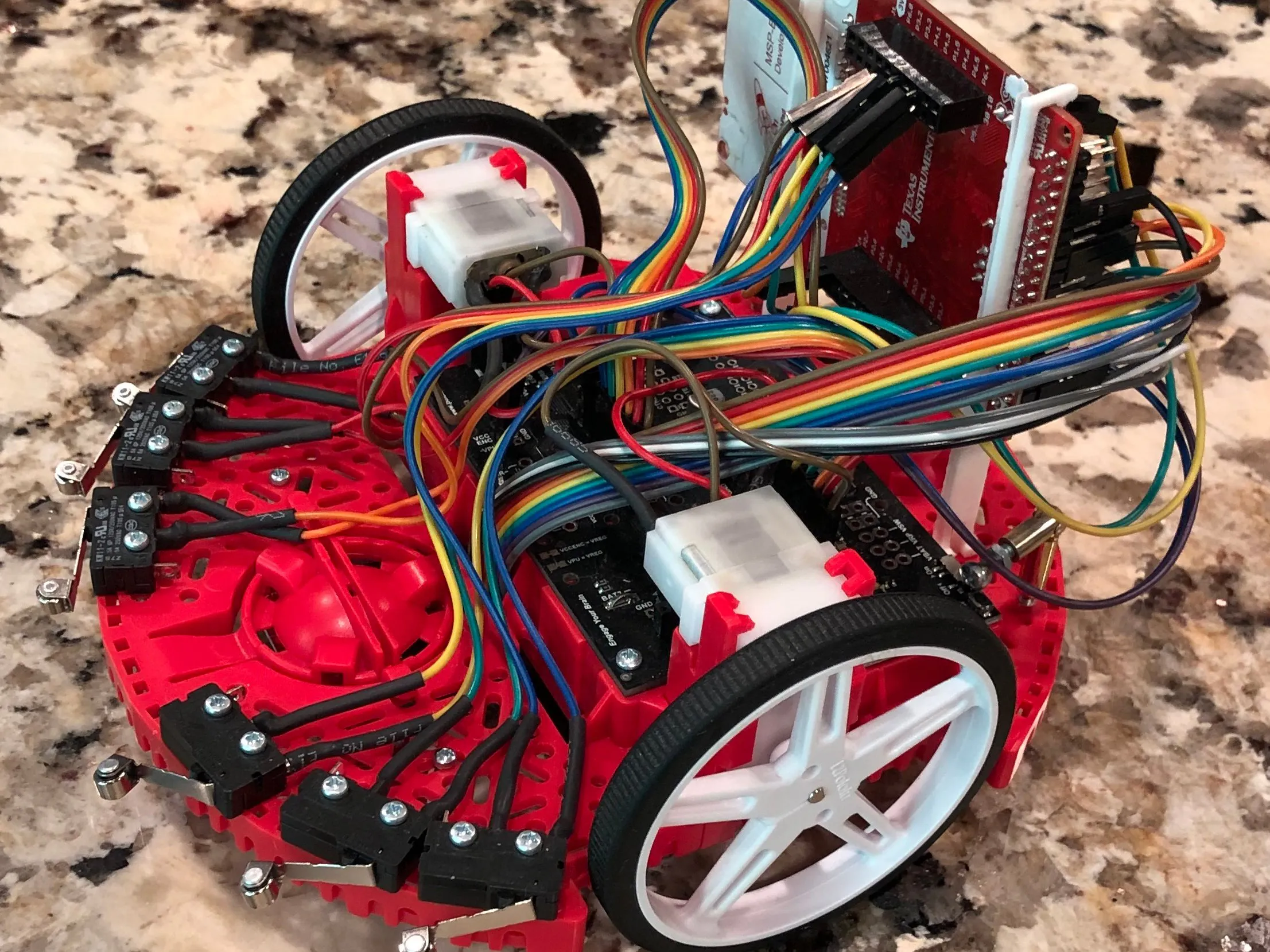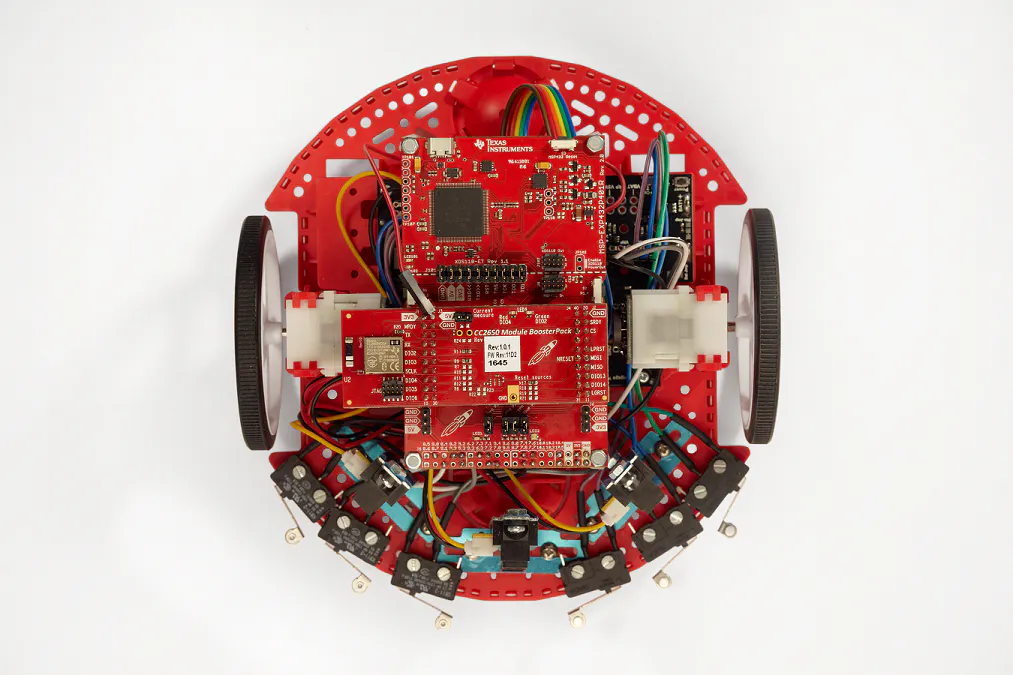Published

TI-RSLK Build Workshop Instructions

Instructions for assembling the TI-RSLK Basic version of the kit during a TI-hosted workshop.

IntermediateProtip4 hours3,842Things used in this project

Hardware componentsTexas Instruments TI Robotics System Learning Kit TI-RSLK
×1
 TE Connectivity Crimp connectors 63705-1
×1

Software apps and online servicesTexas Instruments Energia

Code

RSLK_bump_switch_demo_WallPong Code

Arduino
const int bump_sw_pin1 = 24; // P4.0
const int bump_sw_pin2 = 25; // P4.2
const int bump_sw_pin3 = 6; // P4.3
const int bump_sw_pin4 = 27; // P4.5
const int bump_sw_pin5 = 8; // P4.6
const int bump_sw_pin6 = 28; // P4.7

const int left_slp_pin = 31;  // P3.7
const int left_dir_pin = 14;  // P1.7
const int left_pwm_pin = 40;  // P2.7
const int right_slp_pin = 11;  // P3.6
const int right_dir_pin = 15;  // P1.6
const int right_pwm_pin = 39;  // P2.6

void setup() {
Serial.begin(115200);
Serial.println("Booting.....");
pinMode(bump_sw_pin1, INPUT_PULLUP);
pinMode(bump_sw_pin2, INPUT_PULLUP);
pinMode(bump_sw_pin3, INPUT_PULLUP);
pinMode(bump_sw_pin4, INPUT_PULLUP);
pinMode(bump_sw_pin5, INPUT_PULLUP);
pinMode(bump_sw_pin6, INPUT_PULLUP);

pinMode(left_slp_pin, OUTPUT);      // sets the digital pin as output
pinMode(left_dir_pin, OUTPUT);      // sets the digital pin as output
pinMode(left_pwm_pin, OUTPUT);      // sets the digital pin as output

pinMode(right_slp_pin, OUTPUT);      // sets the digital pin as output
pinMode(right_dir_pin, OUTPUT);      // sets the digital pin as output
pinMode(right_pwm_pin, OUTPUT);      // sets the digital pin as output

analogWrite(left_pwm_pin, 125);
digitalWrite(left_slp_pin, LOW);
digitalWrite(left_dir_pin, LOW);

analogWrite(right_pwm_pin, 125);
digitalWrite(right_slp_pin, LOW);
digitalWrite(right_dir_pin, LOW);

turnOnMotor();
setLeftMotorSpeed(23);
setRightMotorSpeed(25);
runMotorForward();
}

void loop() {
Serial.println("Pin 1 Low");
setLeftMotorSpeed(50);
setRightMotorSpeed(0);
runMotorBackward();
delay(200);
setLeftMotorSpeed(23);
setRightMotorSpeed(25);
runMotorForward();
} else {
Serial.println("Pin 1 High");
}

Serial.println("Pin 2 Low");
setLeftMotorSpeed(50);
setRightMotorSpeed(0);
runMotorBackward();
delay(400);
setLeftMotorSpeed(23);
setRightMotorSpeed(25);
runMotorForward();
} else {
Serial.println("Pin 2 High");
}

Serial.println("Pin 3 Low");
setLeftMotorSpeed(50);
setRightMotorSpeed(0);
runMotorBackward();
delay(600);
setLeftMotorSpeed(23);
setRightMotorSpeed(25);
runMotorForward();
} else {
Serial.println("Pin 3 High");
}

Serial.println("Pin 4 Low");
setLeftMotorSpeed(0);
setRightMotorSpeed(50);
runMotorBackward();
delay(600);
setLeftMotorSpeed(23);
setRightMotorSpeed(25);
runMotorForward();
} else {
Serial.println("Pin 4 High");
}

Serial.println("Pin 5 Low");
setLeftMotorSpeed(0);
setRightMotorSpeed(50);
runMotorBackward();
delay(400);
setLeftMotorSpeed(23);
setRightMotorSpeed(25);
runMotorForward();
} else {
Serial.println("Pin 5 High");
}

Serial.println("Pin 6 Low");
setLeftMotorSpeed(0);
setRightMotorSpeed(50);
runMotorBackward();
delay(200);
setLeftMotorSpeed(23);
setRightMotorSpeed(25);
runMotorForward();
} else {
Serial.println("Pin 6 High");
}
delay(200);
}

void setLeftMotorSpeed(int speed) {
int val = map(speed, 0, 100, 0, 255);
analogWrite(left_pwm_pin, val);
}

void setRightMotorSpeed(int speed) {
int val = map(speed, 0, 100, 0, 255);
analogWrite(right_pwm_pin, val);
}

void turnOnMotor() {
digitalWrite(left_slp_pin, HIGH);
digitalWrite(right_slp_pin, HIGH);
}

void turnOffMotor() {
digitalWrite(left_slp_pin, LOW);
digitalWrite(right_slp_pin, LOW);
}

void runMotorForward() {
digitalWrite(left_dir_pin, LOW);
digitalWrite(right_dir_pin, LOW);
}

void runMotorBackward() {
digitalWrite(left_dir_pin, HIGH);
digitalWrite(right_dir_pin, HIGH);
}

Line_Follower_Energia_Code

Arduino
//==================================================================
//Below shows how the line sensor pins are mapped to the MSP-EXP432P401R
#define LS_PIN_IR  61 // <- Energia Pin #   Launchpad Pin -> P5.3
#define LS_PIN_1  65 // <- Energia Pin #   Launchpad Pin -> P7.0
#define LS_PIN_2  48 // <- Energia Pin #   Launchpad Pin -> P7.1
#define LS_PIN_3  64 // <- Energia Pin #   Launchpad Pin -> P7.2
#define LS_PIN_4  47 // <- Energia Pin #   Launchpad Pin -> P7.3
#define LS_PIN_5  52 // <- Energia Pin #   Launchpad Pin -> P7.4
#define LS_PIN_6  68 // <- Energia Pin #   Launchpad Pin -> P7.5
#define LS_PIN_7  53 // <- Energia Pin #   Launchpad Pin -> P7.6
#define LS_PIN_8  69 // <- Energia Pin #   Launchpad Pin -> P7.7

//Below shows Motor Driver pins
#define MOTOR_L_SLP_PIN 31  // <- Energia Pin #   Launchpad Pin -> P3.7
#define MOTOR_L_DIR_PIN 14  // <- Energia Pin #   Launchpad Pin -> P1.7
#define MOTOR_L_PWM_PIN 40  // <- Energia Pin #   Launchpad Pin -> P2.7
#define MOTOR_R_SLP_PIN 11  // <- Energia Pin #   Launchpad Pin -> P3.6
#define MOTOR_R_DIR_PIN 15  // <- Energia Pin #   Launchpad Pin -> P1.6
#define MOTOR_R_PWM_PIN 39  // <- Energia Pin #   Launchpad Pin -> P2.6

void setup() {
//Initialize Line Sensors:
pinMode(PUSH2, INPUT_PULLUP);  //Right button on LaunchPad

//setup() From Romi_Motor_Power.cpp
//this setup() should be transferred to Main Example Code
//Initialize Motors:
//setup()
pinMode(MOTOR_L_SLP_PIN, OUTPUT);
pinMode(MOTOR_L_DIR_PIN, OUTPUT);
pinMode(MOTOR_L_PWM_PIN, OUTPUT);
pinMode(MOTOR_R_SLP_PIN, OUTPUT);
pinMode(MOTOR_R_DIR_PIN, OUTPUT);
pinMode(MOTOR_R_PWM_PIN, OUTPUT);
analogWrite(MOTOR_L_PWM_PIN, 0);      //speed = 0
analogWrite(MOTOR_R_PWM_PIN, 0);      //speed = 0
digitalWrite(MOTOR_L_SLP_PIN, LOW);   //put motor to sleep
digitalWrite(MOTOR_R_SLP_PIN, LOW);   //put motor to sleep

//Initialize Line Following Sensor:
pinMode(LS_PIN_IR, OUTPUT);
pinMode(LS_PIN_1, OUTPUT);
pinMode(LS_PIN_2, OUTPUT);
pinMode(LS_PIN_3, OUTPUT);
pinMode(LS_PIN_4, OUTPUT);
pinMode(LS_PIN_5, OUTPUT);
pinMode(LS_PIN_6, OUTPUT);
pinMode(LS_PIN_7, OUTPUT);
pinMode(LS_PIN_8, OUTPUT);

Serial.begin(9600);
Serial.println("starting...");
}

void loop() {
//=====================================================================
//motor pin variables
uint8_t slp_pin_L = MOTOR_L_SLP_PIN;
uint8_t dir_pin_L = MOTOR_L_DIR_PIN;
uint8_t pwm_pin_L = MOTOR_L_PWM_PIN;
uint8_t motor_dir_L = 0;
uint8_t slp_pin_R = MOTOR_R_SLP_PIN;
uint8_t dir_pin_R = MOTOR_R_DIR_PIN;
uint8_t pwm_pin_R = MOTOR_R_PWM_PIN;
uint8_t motor_dir_R = 0;
//line following sensor variables
digitalWrite(LS_PIN_IR, HIGH);
digitalWrite(LS_PIN_1, HIGH);
digitalWrite(LS_PIN_2, HIGH);
digitalWrite(LS_PIN_3, HIGH);
digitalWrite(LS_PIN_4, HIGH);
digitalWrite(LS_PIN_5, HIGH);
digitalWrite(LS_PIN_6, HIGH);
digitalWrite(LS_PIN_7, HIGH);
digitalWrite(LS_PIN_8, HIGH);

//wait until button is pressed to start robot motors

//enable motors
digitalWrite(slp_pin_L, HIGH);
digitalWrite(slp_pin_R, HIGH);

//set motors to go forward
digitalWrite(dir_pin_L, LOW);
digitalWrite(dir_pin_R, LOW);

//test code for motors
/*for(int i=20; i<255; i++){
analogWrite(pwm_pin_L, i);  //ramp motors from 0 to full speed
analogWrite(pwm_pin_R, i);  //ramp motors from 0 to full speed
delay(50);
}*/

bool pin1 = 1;
bool pin2 = 1;
bool pin3 = 1;
bool pin4 = 1;
bool pin5 = 1;
bool pin6 = 1;
bool pin7 = 1;
bool pin8 = 1;

//======================================================================
//THIS AREA IS JUST FOR TESTING THE LINE SENSOR SENSITIVITY AND LIGHTING
//======================================================================
/*
while(true)
{
Serial.println("Testing time for line following sensor sensitivity:");

for(int i=0; i<100; i+=1){  //set how much time to test for
digitalWrite(LS_PIN_IR, LOW);
pinMode(LS_PIN_1, INPUT);
pinMode(LS_PIN_2, INPUT);
pinMode(LS_PIN_3, INPUT);
pinMode(LS_PIN_4, INPUT);
pinMode(LS_PIN_5, INPUT);
pinMode(LS_PIN_6, INPUT);
pinMode(LS_PIN_7, INPUT);
pinMode(LS_PIN_8, INPUT);
delay(i);
delay(10);
Serial.print("Time = ");
Serial.print(i);
Serial.println("ms");
Serial.print(pin8);
Serial.print(pin7);
Serial.print(pin6);
Serial.print(pin5);
Serial.print(pin4);
Serial.print(pin3);
Serial.print(pin2);
Serial.println(pin1);

//CHARGE BACK UP AND GET READY TO SENSE AGAIN
pinMode(LS_PIN_1, OUTPUT);
pinMode(LS_PIN_2, OUTPUT);
pinMode(LS_PIN_3, OUTPUT);
pinMode(LS_PIN_4, OUTPUT);
pinMode(LS_PIN_5, OUTPUT);
pinMode(LS_PIN_6, OUTPUT);
pinMode(LS_PIN_7, OUTPUT);
pinMode(LS_PIN_8, OUTPUT);
digitalWrite(LS_PIN_IR, HIGH);
digitalWrite(LS_PIN_1, HIGH);
digitalWrite(LS_PIN_2, HIGH);
digitalWrite(LS_PIN_3, HIGH);
digitalWrite(LS_PIN_4, HIGH);
digitalWrite(LS_PIN_5, HIGH);
digitalWrite(LS_PIN_6, HIGH);
digitalWrite(LS_PIN_7, HIGH);
digitalWrite(LS_PIN_8, HIGH);
delay(100);
}
}
*/
//======================================================================
//THIS AREA IS FOR LINE FOLLOWING APPLICATION
//======================================================================
int time = 10; //ENTER VALUE HERE FROM YOUR SENSITIVITY TESTS
Serial.println("Entering line following mode:");

while(true){
int steering = 128;
digitalWrite(LS_PIN_IR, LOW); //turn off LED
pinMode(LS_PIN_1, INPUT);     //turn on sensors
pinMode(LS_PIN_2, INPUT);
pinMode(LS_PIN_3, INPUT);
pinMode(LS_PIN_4, INPUT);
pinMode(LS_PIN_5, INPUT);
pinMode(LS_PIN_6, INPUT);
pinMode(LS_PIN_7, INPUT);
pinMode(LS_PIN_8, INPUT);
delay(time);                     //USE YOUR SENSITIVITY TEST TO FIGURE OUT THIS VALUE
if (pin1) steering += 64;
if (pin2) steering += 32;
if (pin3) steering += 16;
if (pin4) steering += 8;
if (pin5) steering -= 8;
if (pin6) steering -= 16;
if (pin7) steering -= 32;
if (pin8) steering -= 64;

//CHARGE BACK UP AND GET READY TO SENSE AGAIN
pinMode(LS_PIN_1, OUTPUT);
pinMode(LS_PIN_2, OUTPUT);
pinMode(LS_PIN_3, OUTPUT);
pinMode(LS_PIN_4, OUTPUT);
pinMode(LS_PIN_5, OUTPUT);
pinMode(LS_PIN_6, OUTPUT);
pinMode(LS_PIN_7, OUTPUT);
pinMode(LS_PIN_8, OUTPUT);
digitalWrite(LS_PIN_IR, HIGH);
digitalWrite(LS_PIN_1, HIGH);
digitalWrite(LS_PIN_2, HIGH);
digitalWrite(LS_PIN_3, HIGH);
digitalWrite(LS_PIN_4, HIGH);
digitalWrite(LS_PIN_5, HIGH);
digitalWrite(LS_PIN_6, HIGH);
digitalWrite(LS_PIN_7, HIGH);
digitalWrite(LS_PIN_8, HIGH);

//Serial.println(steering);
if (steering > 205) analogWrite(pwm_pin_L, 60);
else if (steering > 175) analogWrite(pwm_pin_L, 40);
else if (steering > 150) analogWrite(pwm_pin_L, 20);
else analogWrite(pwm_pin_L, 0);

if (steering < 50) analogWrite(pwm_pin_R, 60);  //ramp motors from 0 to full speed
else if (steering < 80) analogWrite(pwm_pin_R, 40);
else if (steering < 105) analogWrite(pwm_pin_R, 20);
else analogWrite(pwm_pin_R, 0);

if (steering >= 106 && steering <= 149){
analogWrite(pwm_pin_L, 40);
analogWrite(pwm_pin_R, 40);
}
} //END OF WHILE() LOOP
} //END OF LOOP()

Credits

4 projects • 9 followers
Texas Instruments University Marketing Manager for the US West

Mark Easley

60 projects • 124 followers

Mark Easley

0 projects • 2 followers

Ayesha Mayhugh

0 projects • 0 followers

Ayesha Mayhugh

0 projects • 0 followers

Franklin Cooper Jr.

4 projects • 13 followers